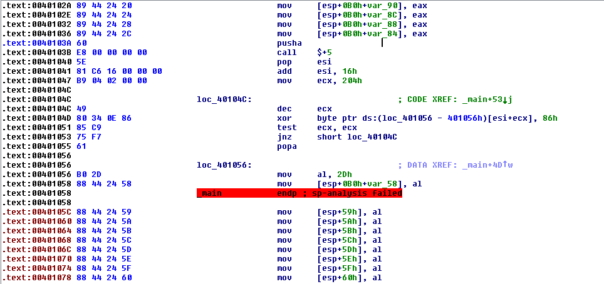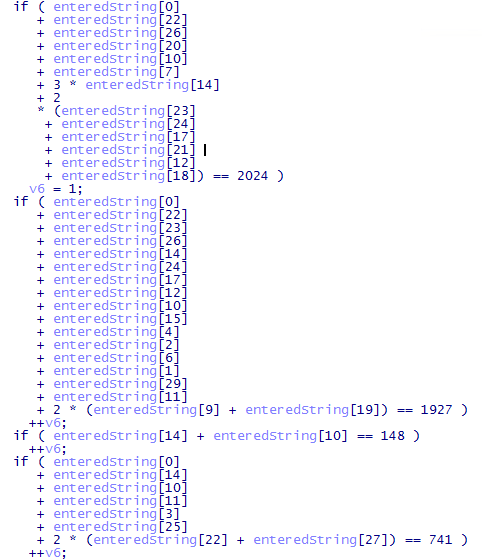02 Nov 2015

Yesterday Extreme Coders posted a small crackme on Tuts4You. It's quite an easy one but solving it would require either lots of typing or some clever automation. Of course, being lazy I went for the automation route! smile

### Initial analysis

My preferred way is doing static analysis in IDA and - when necessary do dynamic analysis using OllyDbg. So, here is how it looks like in IDA:As you can see, parts of code have been encrypted. 102 parts of code, to be exact. smile

### Decrypt the code

Since there is a lot of code that's encrypted, I need to automate decryption somehow. IDA scripting to the rescue!

There's not much to comment. There's a big loop that's looking for the pattern of the decryption code. Then it extracts information about encrypted code address, size and used encryption key. Finally it decrypts the code.

Note - when you're patching binary data in IDA, it's always better to force IDA to reanalyze the affected fragment. I didn't do that here because changing end of _main() will force analysis automatically.

After decryption the code looks much better:Well, it's better, but it still kinda sucks. We have 100 checks like this:

So, we're solving system of 100 linear equations with 32 variables. Great! Who wants to write down these equations based on disassembly and then solve them manually? Not me!

### Decompile the code

Let's see if we can somehow make the problem easier for us. Hexrays decompiler provides nice output but it still needs a lot of cleanup:Basically, the code responsible for encryption/decryption of checks is getting into our way.

Another IDA script to the rescue:

I took the previous script and modified it a bit. Now it finds both encryption and decryption loops and just nops them out. It also forces IDA to reanalyze the patched region - it's very important because otherwise IDA lost track of correct stack pointer and decompiled code was wrong.

Quick changes in Hexrays plugin options to use decimal radix and the decompiled code looks great!### Text editor magic

Beginning reversers commonly underestimate power of text editors. Sure, the Hexrays output we got is readable, but it's not really suitable for any sort of automated processing.

First, let's get rid of all extra spaces. Replace " " (2 spaces) with " " (one space). Repeat until no more matches. Now it looks like this:

Put each equation on one line. Replace "\r\n +" (new line,space,plus) with " +" (space,plus). Replace "\r\n *" (new line,space,star) with " *" (space,star).

Get rid of those "if". Get rid of "++v6;". Replace "==" with "=".

Finally, rename "enteredString" to "z" and get rid of those "[" and "]"

Congratulations, within one minute you got from ugly decompiled code to well-written system of equations!

### And solve the problem

Nicely written system of equations is pointless, if you can't solve it. Luckily, there's an online solver for that right there! wink Copy-pasting our cleaned system of equations into their webform gives us result:

This system has a unique solution, which is

{ z0 = 102, z1 = 108, z10 = 48, z11 = 108, z12 = 118, z13 = 101, z14 = 100, z15 = 95, z16 = 116, z17 = 104, z18 = 97, z19 = 116, z2 = 97, z20 = 95, z21 = 114, z22 = 49, z23 = 103, z24 = 104, z25 = 116, z26 = 33, z27 = 33, z28 = 33, z29 = 125, z3 = 103, z4 = 123, z5 = 89, z6 = 48, z7 = 117, z8 = 95, z9 = 115 }.

Converting character codes to ANSI string is an equally simple exercice, I'm not gonna bore you with that.

And that's how you solve a crackme with nothing but a few scripts in IDA and a text editor.. wink

## 12 thoughts on “Solving “Find the flag” crackme by Extreme Coders”

1.kao, do you ever sleep? ;)

•More than most people. :) I must get my 8 hours of sleep every night, otherwise I'm virtually useless the next day.

2.Putting the equation in the solver you proposed:

z0 + z22 + z26 + z20 + z10 + z7 + 3 * z14 + 2 * (z23 + z24 + z17 + z21 + z12 + z18) = 2024
z0 + z22 + z23 + z26 + z14 + z24 + z17 + z12 + z10 + z15 + z4 + z2 + z6 + z1 + z29 + z11 + 2 * (z9 + z19) = 1927
z14 + z10 = 148
z0 + z14 + z10 + z11 + z3 + z25 + 2 * (z22 + z27) = 741
z24 + z29 = 229

Gives me:

This system has infinitely many solutions, which are (with parameters ri):

{ z0 = - r9- r8- r7- r6-2 r5-2 r4- r3- r18- r16- r14- r12- r11- r10 +1550, z1 = r8, z10 = 148- r19, z11 = r6, z12 = r14, z14 = r19, z15 = r12, z17 = r16, z18 = r13, z19 = r4, z2 = r10, z20 = r9+ r8+ r6+2 r5+2 r4- r20-2 r19- r18-2 r17- r16-2 r15- r14 -2 r13+ r12+ r11+ r10+326, z21 = r15, z22 = r7, z23 = r18, z24 = r17, z25 = r2, z26 = r3, z27 = r1, z29 = 229- r17, z3 = r9+ r8- r7+2 r5+2 r4+ r3- r2+ r18+ r16+ r14+ r12+ r11+ r10 -2 r1-957, z4 = r11, z6 = r9, z7 = r20, z9 = r5 }.

Do you have any idea what I'm doing wrong?

Greetings

•You have to put in all those 100 equations.

•Guys, thanks for feedback, I updated post to make it more obvious that there are more lines to follow.

3.Tks for your nice write-up :)
I re-write it in my mother language with some details for me and my friends: {hidden link}

Regards,
m4n0w4r

•Hehe, you made quite some effort to both translate the writeup and explain every step in detail. Good work! :)

4.Really great writeup ... thank you!
You really convinced me it's time to start learning IDA scripting ;)

Regards,
Tony

5.So the green triangle from the first column is 0, and the purple triangle from last column is 0xC. Forcing path down to 00402A87 sets the goodboy flag and makes the crackme display congratulations. We are not interested in patching, as that would be too easy. Besides, authors explicitly forbid it.

•What an interesting spam! Or did I miss the point of the post? :D